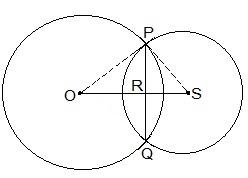Guru

# Two circles of radii 5 cm and 3 cm intersect at two points and the distance between their centres is 4 cm. Find the length of the common chord. Q.1

• 1

Hello sir i want to know the best solution of the question from exercise 10.4 of math of Circles chapter of class 9th give me the best and easy for solving this question how i solve it of question no.1 Two circles of radii 5 cm and 3 cm intersect at two points and the distance between their centers is 4 cm. Find the length of the common chord.

Share

1. Given parameters are:

OP = 5cm

OS = 4cm and

PS = 3cm

Also, PQ = 2PR

Now, suppose RS = x. The diagram for the same is shown below.Consider the ΔPOR,

OP2 = OR2+PR2

⇒ 52 = (4-x)2+PR2

⇒ 25 = 16+x2-8x+PR2

∴ PR2 = 9-x2+8x — (i)

Now consider ΔPRS,

PS2 = PR2+RS2

⇒ 32 = PR2+x2

∴ PR2 = 9-x2 — (ii)

By equating equation (i) and equation (ii) we get,

9 -x2+8x = 9-x2

⇒ 8x = 0

⇒ x = 0

Now, put the value of x in equation (i)

PR2 = 9-02

⇒ PR = 3cm

∴ The length of the cord i.e. PQ = 2PR

So, PQ = 2×3 = 6cm

• 0